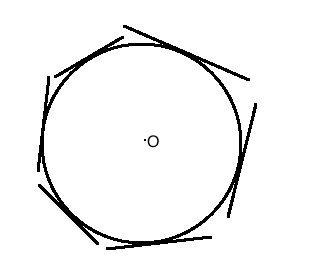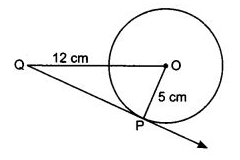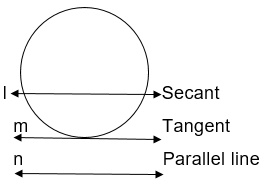Courses

# Notes | EduRev

## Class 10 : Notes | EduRev

The document Notes | EduRev is a part of the Class 10 Course Class 10 Mathematics by VP Classes.
All you need of Class 10 at this link: Class 10

Page No. 209

Q.1. How many tangents can a circle have?
Sol.
A circle can have an infinite number of tangents.Q.2. Fill in the blanks
(i) A tangent to a circle intersects it in .......... point(s).
(ii) A line intersecting a circle in two points is called a ...........
(iii) A circle can have .......... parallel tangents at the most.
(iv) The common point of a tangent to a circle and the circle is called ...........

Sol.
(i) exactly one
(ii) secant
(iii) two
(iv) point of contact.

Q.3. A tangent PQ at a point P of a circle of radius 5 cm meets a line through the centre O at a point Q so that OQ = 12 cm. Length of PQ is:
(a) 12 cm
(b) 13 cm
(c) 8.5 cm

(d) √199 cm

Ans. (d)
Sol.Radius of the circle = 5 cm

OQ = 12 cm

∠OPQ = 900

[The tangent to a circle is perpendicular to the radius through the point of contact]

PQ2 = OQ2 - OP[By Pythagoras theorem]

PQ= 12- 5= 144 - 25 = 199

PQ = √199 cm

Hence correct option is (d)

Q.4. Draw a circle and two lines parallel to a given line such that one is a tangent and the other a secant to the circle.
Sol. A line 'm' is parallel to the given line 'n' and a line 'l' which is secant is parallel to the given line 'n'.Offer running on EduRev: Apply code STAYHOME200 to get INR 200 off on our premium plan EduRev Infinity!

132 docs

,

,

,

,

,

,

,

,

,

,

,

,

,

,

,

,

,

,

,

,

,

;